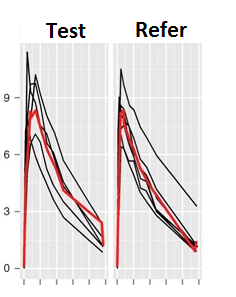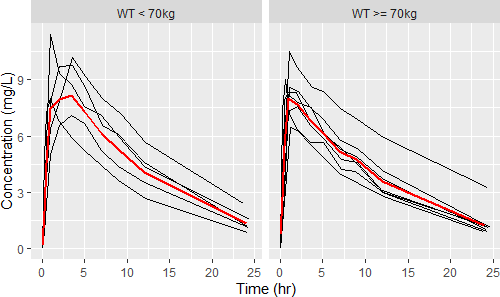Weidson
☆

Brazil,
2021-12-22 18:47
(30 d 02:09 ago)

Posting: # 22709
Views: 339

R script published pubmed site not work [R for BE/BA]

Dear Friends,

I would like to understand why the code bellow is not working. This code I was take out on the page: https://www.ncbi.nlm.nih.gov/pmc/articles/PMC3817376/. Would it be possible for any BEBAC member to adapt it to work? I need of the a graphics tool (for example: ggplot2) that to make graphics dinamic way as we can make at Phoenix WinNonlin.

(ggplot2)
library(nlme)

ggplot(data=Theoph, aes(x=Time, y=conc, group=Subject)) + geom_line() + labs(x=“Time (hr)”, y=“Concentration (mg/L)”)

p <- ggplot(data=Theoph, aes(x=Time, y=conc, group=Subject)) + geom_line() + labs(x=“Time (hr)”, y=“Concentration (mg/L)”) + stat_summary(fun.y=median, geom=“line”,aes(x=ntpd, y=conc, group=1), color=“red”, size=1)

print(p) # “p” is a ggplot object

# create a flag for body weight

Theoph$WT <- ifelse(Theoph$Wt<70, “WT < 70kg”, “WT >= 70kg”)
p + facet_grid(.~WT)""t>

In true, I need of a dinamic code (for example: ggplot2) for to plot a graphic of Individual plasma concentration profiles sorted by treatment and period with whih the mean. Example:mittyri
★★

Russia,
2021-12-22 21:34
(29 d 23:22 ago)

@ Weidson
Posting: # 22710
Views: 275

use Supplementary for the code

Hi Weidson,

» I would like to understand why the code bellow is not working.
There are 2 problems:
- the code in the paper cannot work due to different quotation marks used (left and right), they should be substituted to simple quotations (" or ')
- there are some parts of the code not presented in the paper

So the best way is to use supplementary material as suggested
Below you can find R code from there. Note that the article is old, so some ggplot2 functions are deprecated, but still working.

library(nlme)

#Figure 1(d)
ggplot(data=Theoph, aes(x=Time, y=conc, group=Subject)) +
geom_line() +
labs(x="Time (hr)", y="Concentration (mg/L)")

##################################################################################
## we need some data manipulation for Figure 1(e) and Figure (f)
## below code is how to calculate approximate ntpd (nominal post time dose)
## "ntpd" is used for summarizing conc data (calculate mean at each time point)
## create body weight category for <70 kg or >=70 kg
##################################################################################

#--create a cut (time intervals)
Theoph$cut <- cut(Theoph$Time, breaks=c(-0.1,0,1,1.5, 2,3,4,6,8,12,16,20,24))

#--make sure each time point has reasonable data
table(Theoph$cut) #--calcuate approximate ntpd library(plyr) tab <- ddply(Theoph, .(cut), summarize, ntpd=round(mean(Time, na.rm=T),2)) #--merge ntpd into Theoph data Theoph <- merge(Theoph, tab, by=c("cut"), all.x=T) #--sort the data by Subject and Time, select only nessesary columns Theoph <- Theoph[order(Theoph$Subject, Theoph$Time),c("Subject","Wt","Dose","Time","conc","ntpd")] #head(Theoph) #--create body weight category for <70 kg or >=70 kg for Figure 1(f) Theoph$WT <- ifelse(Theoph$Wt<70, "WT < 70kg", "WT >= 70kg") #--end of data manipulation ################################################################################## # Figure 1(e) ggplot(data=Theoph, aes(x=Time, y=conc, group=Subject)) + geom_line() + labs(x="Time (hr)", y="Concentration (mg/L)") + stat_summary(fun.y = mean, geom = "line", aes(x=ntpd, group = 1), color = "red", size = 1) #--save as a ggplot object "p" p <- ggplot(data=Theoph, aes(x=Time, y=conc, group=Subject)) + geom_line() + labs(x="Time (hr)", y="Concentration (mg/L)") + stat_summary(fun.y = mean, geom = "line", aes(x=ntpd, group = 1), color = "red", size = 1) print(p) # Figure 1(f) p + facet_grid(.~WT) plot1 <- p + facet_grid(.~WT) #save for later use (section "Multiple Plots on One Page") Kind regards, Mittyri Helmut ★★★Vienna, Austria, 2021-12-22 22:44 (29 d 22:12 ago) @ Weidson Posting: # 22712 Views: 273 Supp. material + corrections Hi Weidson, a couple of problems: • Typographic quotation marks “ ” – only simple double " " or single quotes ' ' work in any programming language. • Theoph is no more in package nlme (the ChangeLog doesn’t tell when it was removed). Now it is in package datasets, which is automatically attached. • You need library(plyr) and a script given in the Supplementary material to create ‘nominal’ times (the ntpd column of the data.frame in the Theoph-list). IMHO, the last cut of 24 is unfortunate, try sort(unique(Theoph$ntpd))
with the original code. My quick-and-dirty correction below.
• Warning message:
fun.y is deprecated. Use fun instead.

Such a warning inmeans, that a function or its syntax is still supported but can be removed in the next release of a package without further notice. Hence, never ignore such a warning – always modify the script accordingly.

» […] I need of a dinamic code for to plot a graphic of Individual plasma concentration profiles sorted by treatment and period with whih the mean.

In practice use the nominal time for grouping.

library(ggplot2)
# library(nlme) # not needed
library(plyr)
geomean <- function(x) {
if (sum(x <= 0) > 0) x[x <= 0] <- NA
return(exp(mean(log(x), na.rm = TRUE)))
}

Theoph     <- datasets::Theoph # only needed if you re-run the code
# this is a workaround since the nominal times are unknown
Theoph$cut <- cut(Theoph$Time,
breaks = c(-0.1, 0, 1, 1.5, 2, 3,
4, 6, 8, 12, 16, 20, 25)) # original 24 is not a good idea
tab        <- ddply(Theoph, .(cut), summarize,
ntpd = round(mean(Time, na.rm = TRUE), 2))
Theoph     <- merge(Theoph, tab, by = c("cut"), all.x = TRUE)
Theoph     <- Theoph[order(Theoph$Subject, Theoph$Time),
c("Subject", "ntpd", "Time", "conc", "Dose", "Wt")]
Theoph$WT <- ifelse (Theoph$Wt < 70, "WT < 70kg", "WT >= 70kg")
p <- ggplot(data = Theoph, aes(x = Time, y = conc, group = Subject)) +
geom_line() +
labs(x = "Time (hr)", y = "Concentration (mg/L)") +
stat_summary(fun = geomean, geom = "line", # original: fun.y = mean
aes(x = ntpd, y = conc, group = 1),
color = "red", size = 1)
p + facet_grid(. ~ WT)However, my workaround is also not ideal. It resolves the issue at the last time point but there are still different concentrations aggregated to the same time point in some subjects, e.g.,

print(Theoph[Theoph$Subject == 8 & Theoph$Time <= 1, 1:4], row.names = FALSE)
Subject ntpd Time conc
8 0.00 0.00 0.00
8 0.52
0.25 3.05
8 0.52
0.52 3.05
8 0.52
0.98 7.31

I couldn’t find the original data set of Robert Upton anywhere. I made an educated guess based on ‘Study D’,1,2 where the weight range of twelve subjects was reported with 55–86 kg. The dose was 320 mg and what is given in Theoph$Dose is actually Dose/kg. After more detective work based on Table I: The product was Slophyllin aqueous syrup (Dooner Laboratories), 80 mg theophylline per 15-ml dose. Theoph <- datasets::Theoph Theoph <- cbind(Theoph, ntpd = c(0, 0.25, 0.5, 1, 2, 3.5, 5, 7, 9, 12, 24)) # continue with Theoph$WT <- ...PS: The median in your script is fine. If prefer the geometric mean. Anything is better than the arith­me­tic mean in the original script.PPS: Arghl! Mittyri was faster.1. Upton RA, Sansom L, Guentert TW, Powell JR, Thiercellin J-F, Shah VP, Coates PE, Riegelman S. Evaluation of the Absorption from 15 Commercial Theophylline Products Indicating Deficiencies in Currently Applied Bio­avail­ability Criteria. J Pharmacokin Biopharm. 1980; 8(3): 229–42. doi:10.1007/BF01059644.
2. Interesting: The linear-up / log-down trapezoidal rule was used and $$\small{AUC_{0-\infty}=AUC_{0-\textrm{tlast}}+\frac{\hat{C}_\textrm{tlast}}{k}}$$, i.e., the estimated last concentration in extrapolation. I like that. Furthermore, PK was linear up to 500 mg and hence, additionally $$\small{AUC\times k}$$ was compared (recall this thread).
• […] the assumption of constant clearance in the individual between the occasions of receiving the standard and the test dose is suspect for theophylline. […] If there is evidence that the clearance but not the volume of distribution varies in the individual, the $$\small{AUC\times k}$$ can be used to gain a more precise index of bioavailability than obtainable from $$\small{AUC}$$ alone.

Confirmed, esp. for $$\small{AUC_{0-\infty}}$$:
$$\small{\begin{array}{lc} \textrm{PK metric} & CV_\textrm{geom}\,\%\\\hline AUC_{0-\textrm{tlast}} & {\color{Red} {22.53\%}}\\ AUC_{0-\textrm{tlast}} \times \hat{k} & {\color{Blue} {21.81\%}}\\ AUC_{0-\infty} & {\color{Red} {28.39\%}}\\ AUC_{0-\infty} \times \hat{k} & {\color{Blue} {20.36\%}}\\\hline \end{array}}$$

Dif-tor heh smusma 🖖
Helmut SchützThe quality of responses received is directly proportional to the quality of the question asked. 🚮
Science QuotesIng. Helmut Schütz